# Introduction to Information Retrieval CS 276 Information Retrieval

• Slides: 48Introduction to Information Retrieval CS 276: Information Retrieval and Web Search Christopher Manning and Prabhakar Raghavan Lecture 15: Learning to RankIntroduction to Information Retrieval Sec. 15. 4 Machine learning for IR ranking? § We’ve looked at methods for ranking documents in IR § Cosine similarity, inverse document frequency, pivoted document length normalization, Pagerank, … § We’ve looked at methods for classifying documents using supervised machine learning classifiers § Naïve Bayes, Rocchio, k. NN, SVMs § Surely we can also use machine learning to rank the documents displayed in search results? § Sounds like a good idea § A. k. a. “machine-learned relevance” or “learning to rank”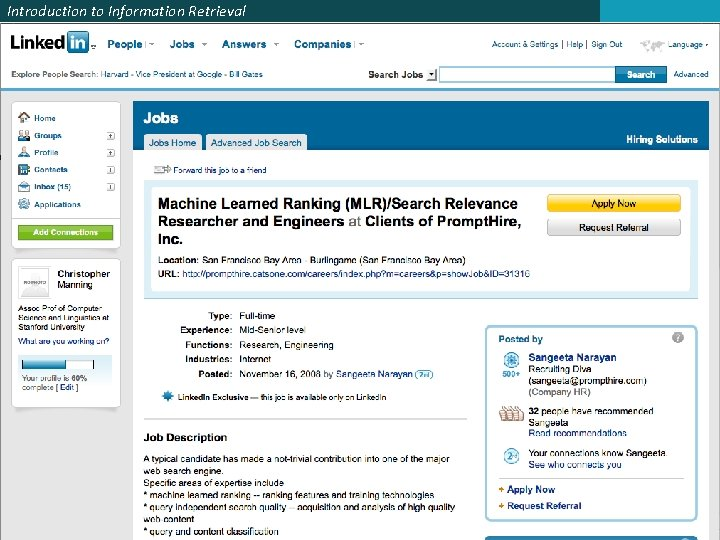Introduction to Information Retrieval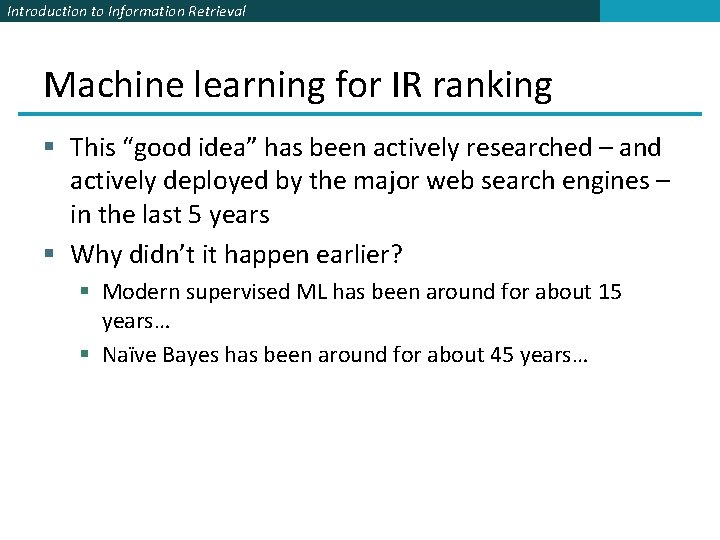Introduction to Information Retrieval Machine learning for IR ranking § This “good idea” has been actively researched – and actively deployed by the major web search engines – in the last 5 years § Why didn’t it happen earlier? § Modern supervised ML has been around for about 15 years… § Naïve Bayes has been around for about 45 years…Introduction to Information Retrieval Machine learning for IR ranking § There’s some truth to the fact that the IR community wasn’t very connected to the ML community § But there were a whole bunch of precursors: § Wong, S. K. et al. 1988. Linear structure in information retrieval. SIGIR 1988. § Fuhr, N. 1992. Probabilistic methods in information retrieval. Computer Journal. § Gey, F. C. 1994. Inferring probability of relevance using the method of logistic regression. SIGIR 1994. § Herbrich, R. et al. 2000. Large Margin Rank Boundaries for Ordinal Regression. Advances in Large Margin Classifiers.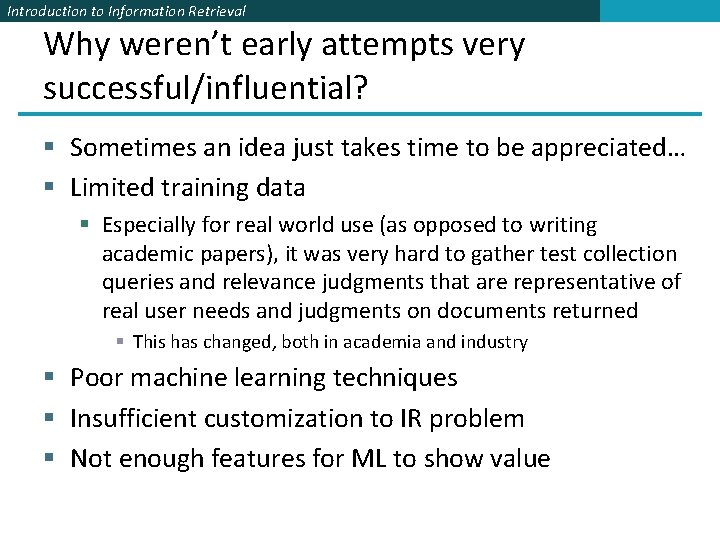Introduction to Information Retrieval Why weren’t early attempts very successful/influential? § Sometimes an idea just takes time to be appreciated… § Limited training data § Especially for real world use (as opposed to writing academic papers), it was very hard to gather test collection queries and relevance judgments that are representative of real user needs and judgments on documents returned § This has changed, both in academia and industry § Poor machine learning techniques § Insufficient customization to IR problem § Not enough features for ML to show value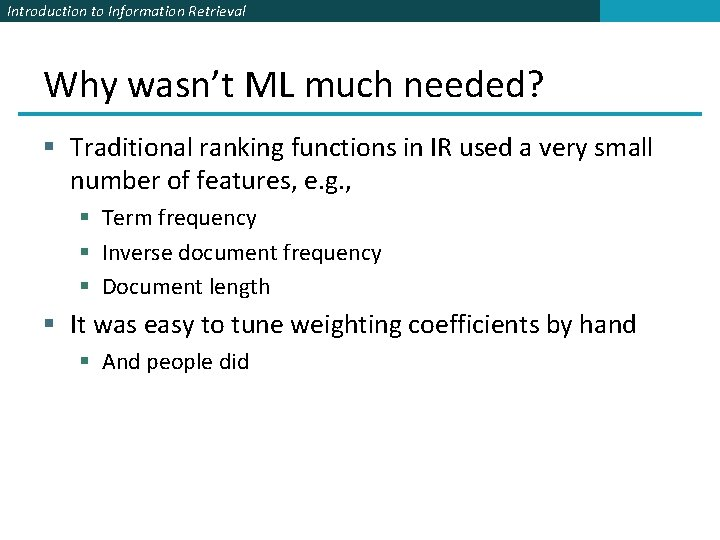Introduction to Information Retrieval Why wasn’t ML much needed? § Traditional ranking functions in IR used a very small number of features, e. g. , § Term frequency § Inverse document frequency § Document length § It was easy to tune weighting coefficients by hand § And people did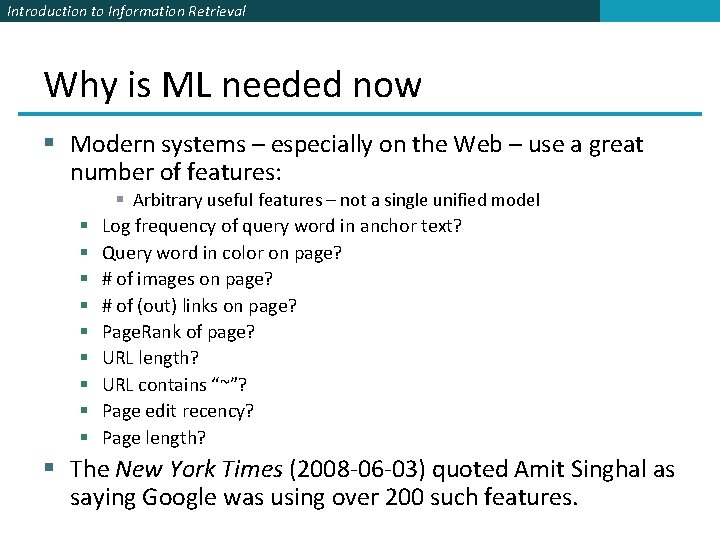Introduction to Information Retrieval Why is ML needed now § Modern systems – especially on the Web – use a great number of features: § § § § § Arbitrary useful features – not a single unified model Log frequency of query word in anchor text? Query word in color on page? # of images on page? # of (out) links on page? Page. Rank of page? URL length? URL contains “~”? Page edit recency? Page length? § The New York Times (2008 -06 -03) quoted Amit Singhal as saying Google was using over 200 such features.Introduction to Information Retrieval Sec. 15. 4. 1 Simple example: Using classification for ad hoc IR § Collect a training corpus of (q, d, r) triples § Relevance r is here binary (but may be multiclass, with 3– 7 values) § Document is represented by a feature vector § x = (α, ω) α is cosine similarity, ω is minimum query window size § ω is the shortest text span that includes all query words § Query term proximity is a very important new weighting factor § Train a machine learning model to predict the class r of a document-query pair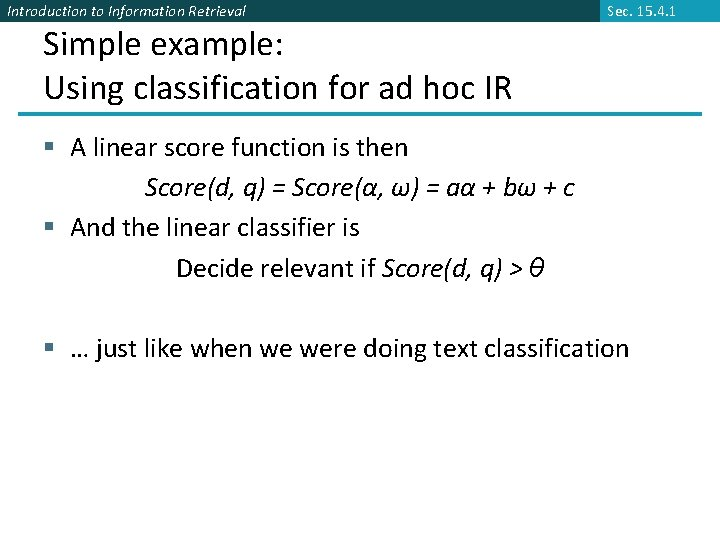Introduction to Information Retrieval Sec. 15. 4. 1 Simple example: Using classification for ad hoc IR § A linear score function is then Score(d, q) = Score(α, ω) = aα + bω + c § And the linear classifier is Decide relevant if Score(d, q) > θ § … just like when we were doing text classificationSec. 15. 4. 1 Introduction to Information Retrieval Simple example: Using classification for ad hoc IR cosine score 0. 05 R 0. 025 R R R R N N 3 N N N 0 2 R N 4 5 Term proximity Decision surfaceIntroduction to Information Retrieval More complex example of using classification for search ranking [Nallapati 2004] § We can generalize this to classifier functions over more features § We can use methods we have seen previously for learning the linear classifier weights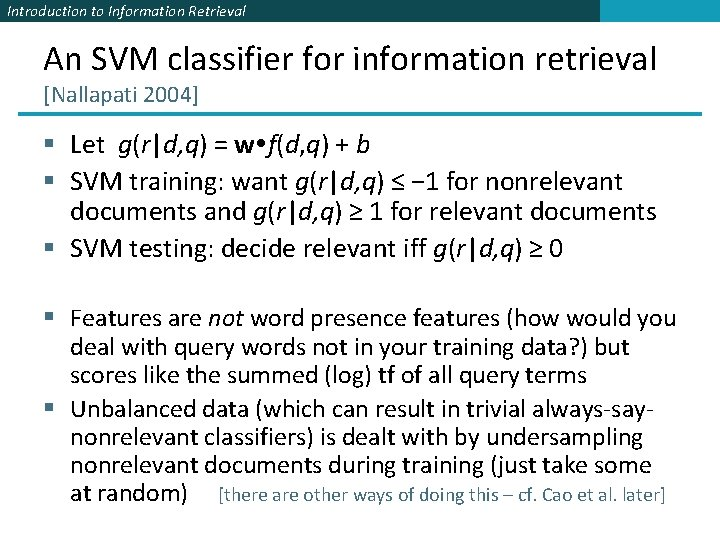Introduction to Information Retrieval An SVM classifier for information retrieval [Nallapati 2004] § Let g(r|d, q) = w f(d, q) + b § SVM training: want g(r|d, q) ≤ − 1 for nonrelevant documents and g(r|d, q) ≥ 1 for relevant documents § SVM testing: decide relevant iff g(r|d, q) ≥ 0 § Features are not word presence features (how would you deal with query words not in your training data? ) but scores like the summed (log) tf of all query terms § Unbalanced data (which can result in trivial always-saynonrelevant classifiers) is dealt with by undersampling nonrelevant documents during training (just take some at random) [there are other ways of doing this – cf. Cao et al. later]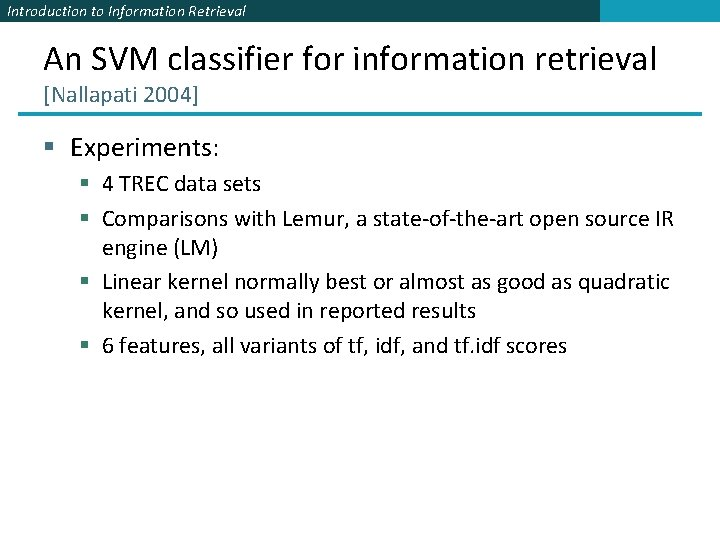Introduction to Information Retrieval An SVM classifier for information retrieval [Nallapati 2004] § Experiments: § 4 TREC data sets § Comparisons with Lemur, a state-of-the-art open source IR engine (LM) § Linear kernel normally best or almost as good as quadratic kernel, and so used in reported results § 6 features, all variants of tf, idf, and tf. idf scores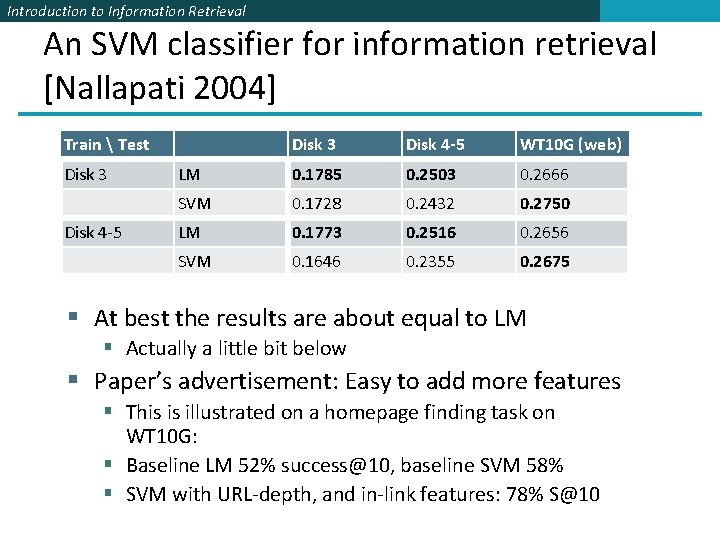Introduction to Information Retrieval An SVM classifier for information retrieval [Nallapati 2004] Train Test Disk 3 Disk 4 -5 WT 10 G (web) LM 0. 1785 0. 2503 0. 2666 SVM 0. 1728 0. 2432 0. 2750 LM 0. 1773 0. 2516 0. 2656 SVM 0. 1646 0. 2355 0. 2675 § At best the results are about equal to LM § Actually a little bit below § Paper’s advertisement: Easy to add more features § This is illustrated on a homepage finding task on WT 10 G: § Baseline LM 52% [email protected], baseline SVM 58% § SVM with URL-depth, and in-link features: 78% [email protected]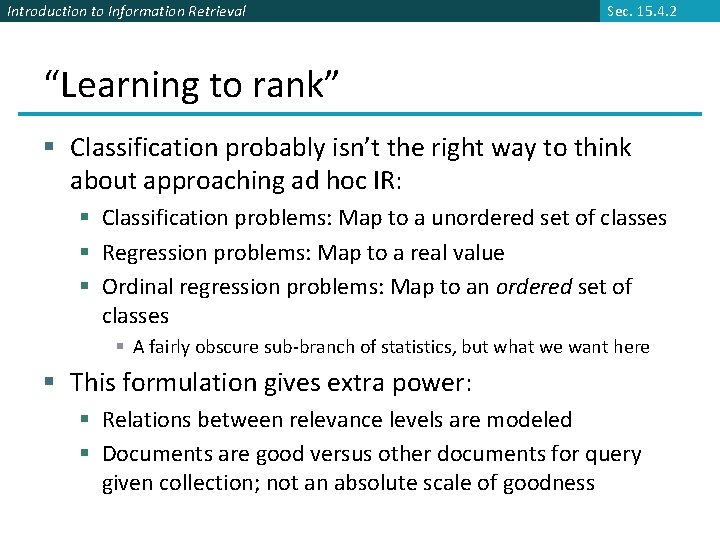Introduction to Information Retrieval Sec. 15. 4. 2 “Learning to rank” § Classification probably isn’t the right way to think about approaching ad hoc IR: § Classification problems: Map to a unordered set of classes § Regression problems: Map to a real value § Ordinal regression problems: Map to an ordered set of classes § A fairly obscure sub-branch of statistics, but what we want here § This formulation gives extra power: § Relations between relevance levels are modeled § Documents are good versus other documents for query given collection; not an absolute scale of goodness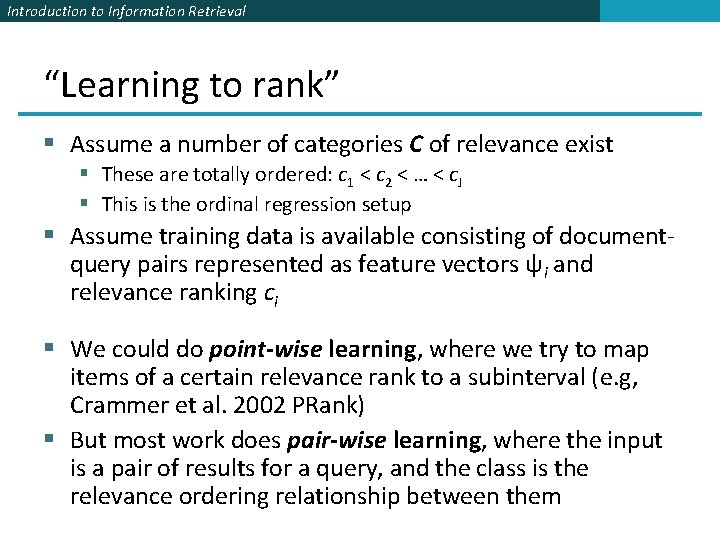Introduction to Information Retrieval “Learning to rank” § Assume a number of categories C of relevance exist § These are totally ordered: c 1 < c 2 < … < c. J § This is the ordinal regression setup § Assume training data is available consisting of documentquery pairs represented as feature vectors ψi and relevance ranking ci § We could do point-wise learning, where we try to map items of a certain relevance rank to a subinterval (e. g, Crammer et al. 2002 PRank) § But most work does pair-wise learning, where the input is a pair of results for a query, and the class is the relevance ordering relationship between themIntroduction to Information Retrieval Point-wise learning § Goal is to learn a threshold to separate each rankIntroduction to Information Retrieval Sec. 15. 4. 2 The Ranking SVM [Herbrich et al. 1999, 2000; Joachims et al. 2002] § Aim is to classify instance pairs as correctly ranked or incorrectly ranked § This turns an ordinal regression problem back into a binary classification problem § We want a ranking function f such that ci > ck iff f(ψi) > f(ψk) § … or at least one that tries to do this with minimal error § Suppose that f is a linear function f(ψi) = w ψi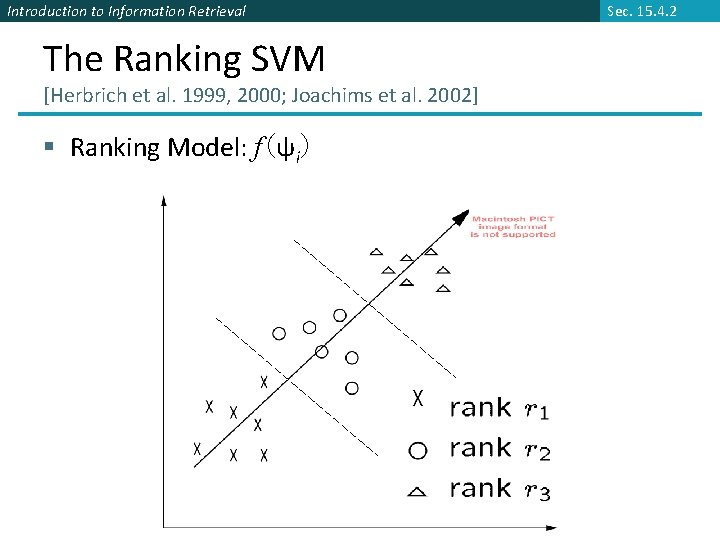Introduction to Information Retrieval The Ranking SVM [Herbrich et al. 1999, 2000; Joachims et al. 2002] § Ranking Model: f(ψi) Sec. 15. 4. 2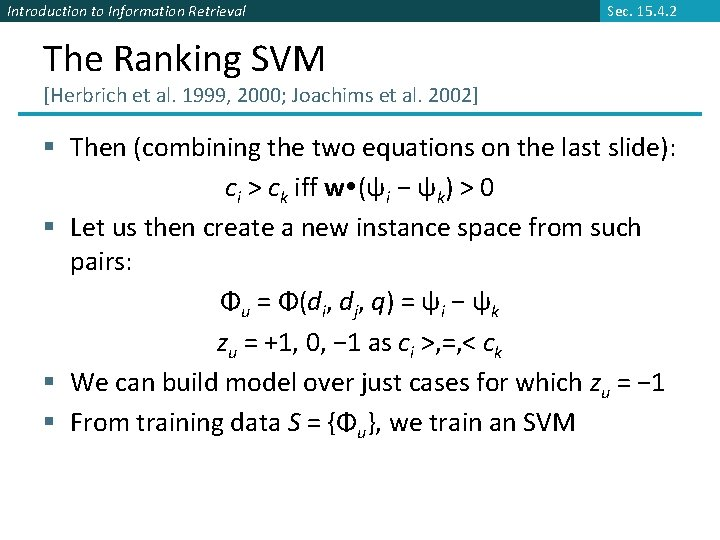Introduction to Information Retrieval Sec. 15. 4. 2 The Ranking SVM [Herbrich et al. 1999, 2000; Joachims et al. 2002] § Then (combining the two equations on the last slide): ci > ck iff w (ψi − ψk) > 0 § Let us then create a new instance space from such pairs: Φu = Φ(di, dj, q) = ψi − ψk zu = +1, 0, − 1 as ci >, =, < ck § We can build model over just cases for which zu = − 1 § From training data S = {Φu}, we train an SVM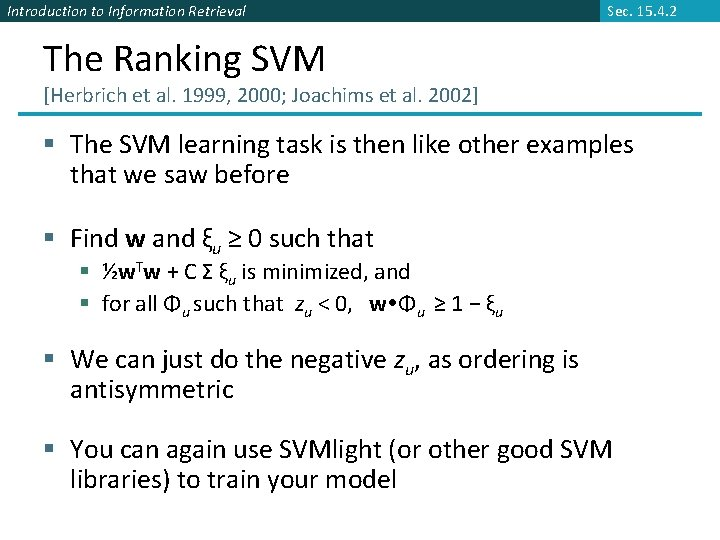Introduction to Information Retrieval Sec. 15. 4. 2 The Ranking SVM [Herbrich et al. 1999, 2000; Joachims et al. 2002] § The SVM learning task is then like other examples that we saw before § Find w and ξu ≥ 0 such that § ½w. Tw + C Σ ξu is minimized, and § for all Φu such that zu < 0, w Φu ≥ 1 − ξu § We can just do the negative zu, as ordering is antisymmetric § You can again use SVMlight (or other good SVM libraries) to train your model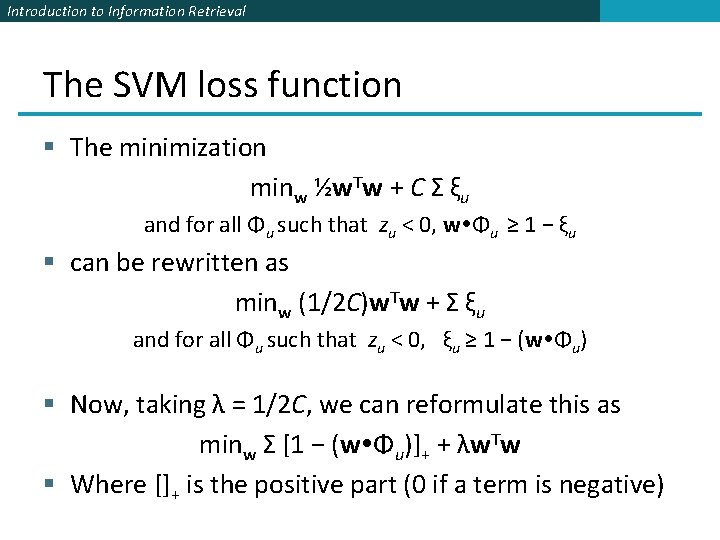Introduction to Information Retrieval The SVM loss function § The minimization minw ½w. Tw + C Σ ξu and for all Φu such that zu < 0, w Φu ≥ 1 − ξu § can be rewritten as minw (1/2 C)w. Tw + Σ ξu and for all Φu such that zu < 0, ξu ≥ 1 − (w Φu) § Now, taking λ = 1/2 C, we can reformulate this as minw Σ [1 − (w Φu)]+ + λw. Tw § Where []+ is the positive part (0 if a term is negative)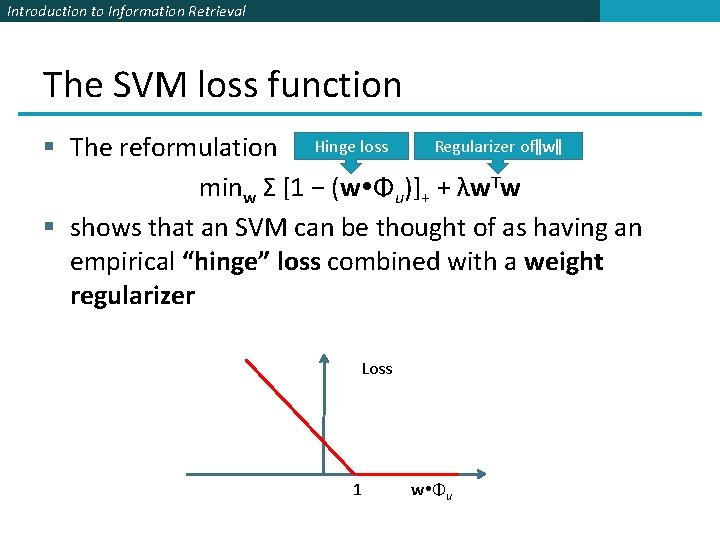Introduction to Information Retrieval The SVM loss function Regularizer of‖w‖ § The reformulation Hinge loss minw Σ [1 − (w Φu)]+ + λw. Tw § shows that an SVM can be thought of as having an empirical “hinge” loss combined with a weight regularizer Loss 1 w Φu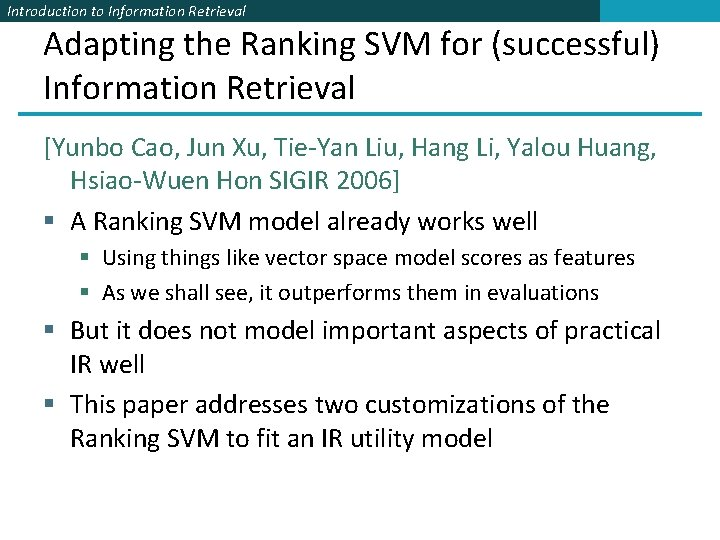Introduction to Information Retrieval Adapting the Ranking SVM for (successful) Information Retrieval [Yunbo Cao, Jun Xu, Tie-Yan Liu, Hang Li, Yalou Huang, Hsiao-Wuen Hon SIGIR 2006] § A Ranking SVM model already works well § Using things like vector space model scores as features § As we shall see, it outperforms them in evaluations § But it does not model important aspects of practical IR well § This paper addresses two customizations of the Ranking SVM to fit an IR utility model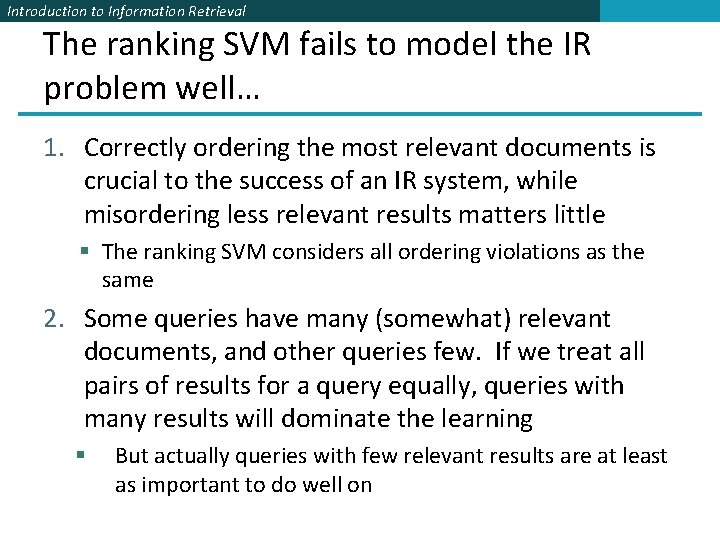Introduction to Information Retrieval The ranking SVM fails to model the IR problem well… 1. Correctly ordering the most relevant documents is crucial to the success of an IR system, while misordering less relevant results matters little § The ranking SVM considers all ordering violations as the same 2. Some queries have many (somewhat) relevant documents, and other queries few. If we treat all pairs of results for a query equally, queries with many results will dominate the learning § But actually queries with few relevant results are at least as important to do well onIntroduction to Information Retrieval Based on the LETOR test collection § From Microsoft Research Asia § An openly available standard test collection with pregenerated features, baselines, and research results for learning to rank § It’s availability has really driven research in this area § OHSUMED, MEDLINE subcollection for IR § § 350, 000 articles 106 queries 16, 140 query-document pairs 3 class judgments: Definitely relevant (DR), Partially Relevant (PR), Non-Relevant (NR) § TREC GOV collection (predecessor of GOV 2, cf. IIR p. 142) § 1 million web pages § 125 queries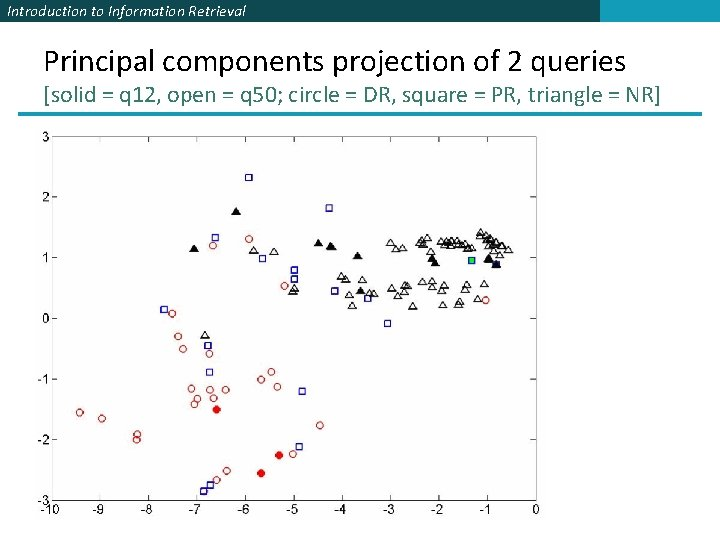Introduction to Information Retrieval Principal components projection of 2 queries [solid = q 12, open = q 50; circle = DR, square = PR, triangle = NR]Introduction to Information Retrieval Ranking scale importance discrepancy [r 3 = Definitely Relevant, r 2 = Partially Relevant, r 1 = Nonrelevant]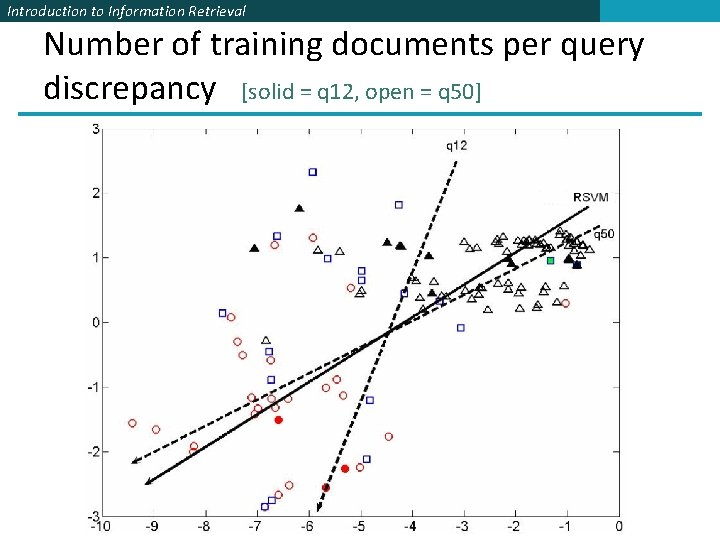Introduction to Information Retrieval Number of training documents per query discrepancy [solid = q 12, open = q 50]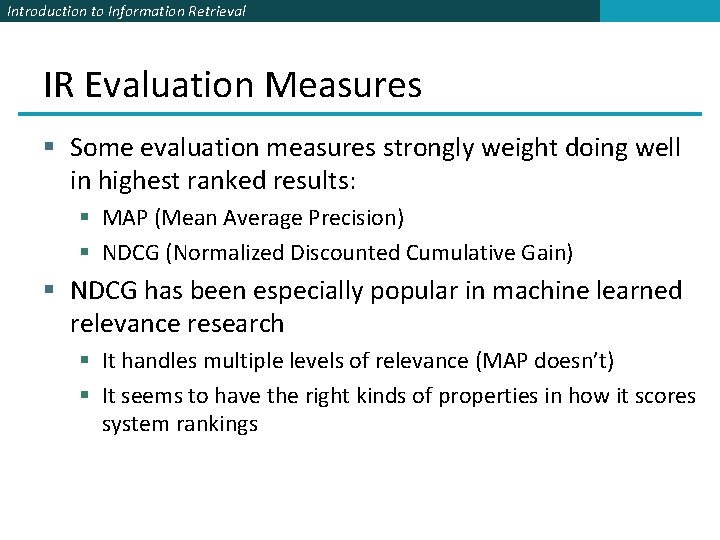Introduction to Information Retrieval IR Evaluation Measures § Some evaluation measures strongly weight doing well in highest ranked results: § MAP (Mean Average Precision) § NDCG (Normalized Discounted Cumulative Gain) § NDCG has been especially popular in machine learned relevance research § It handles multiple levels of relevance (MAP doesn’t) § It seems to have the right kinds of properties in how it scores system rankings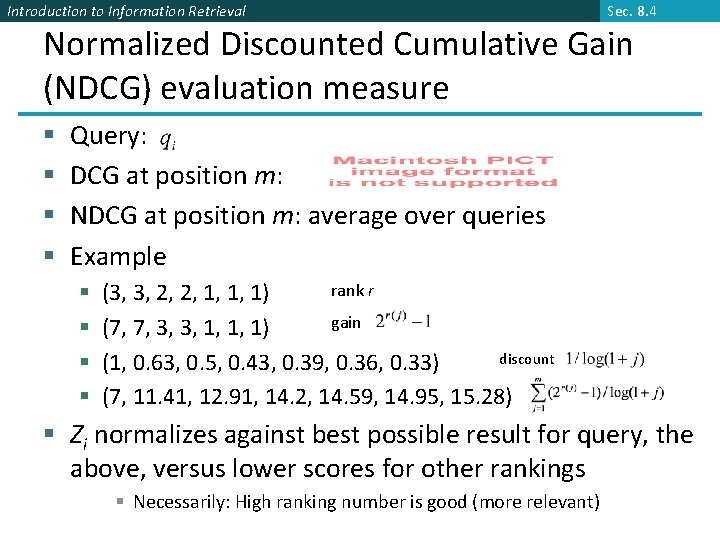Introduction to Information Retrieval Sec. 8. 4 Normalized Discounted Cumulative Gain (NDCG) evaluation measure § § Query: DCG at position m: NDCG at position m: average over queries Example § § rank r (3, 3, 2, 2, 1, 1, 1) gain (7, 7, 3, 3, 1, 1, 1) discount (1, 0. 63, 0. 5, 0. 43, 0. 39, 0. 36, 0. 33) (7, 11. 41, 12. 91, 14. 2, 14. 59, 14. 95, 15. 28) § Zi normalizes against best possible result for query, the above, versus lower scores for other rankings § Necessarily: High ranking number is good (more relevant)Introduction to Information Retrieval Recap: Two Problems with Direct Application of the Ranking SVM § Cost sensitiveness: negative effects of making errors on top ranked documents d: definitely relevant, p: partially relevant, n: not relevant ranking 1: p d p n n ranking 2: d p n n n § Query normalization: number of instance pairs varies according to query q 1: d p p n n q 2: d d p p p n n n q 1 pairs: 2*(d, p) + 4*(d, n) + 8*(p, n) = 14 q 2 pairs: 6*(d, p) + 10*(d, n) + 15*(p, n) = 31Introduction to Information Retrieval These problems are solved with a new Loss function § τ weights for type of rank difference § Estimated empirically from effect on NDCG § μ weights for size of ranked result set § Linearly scaled versus biggest result setIntroduction to Information Retrieval Optimization (Gradient Descent)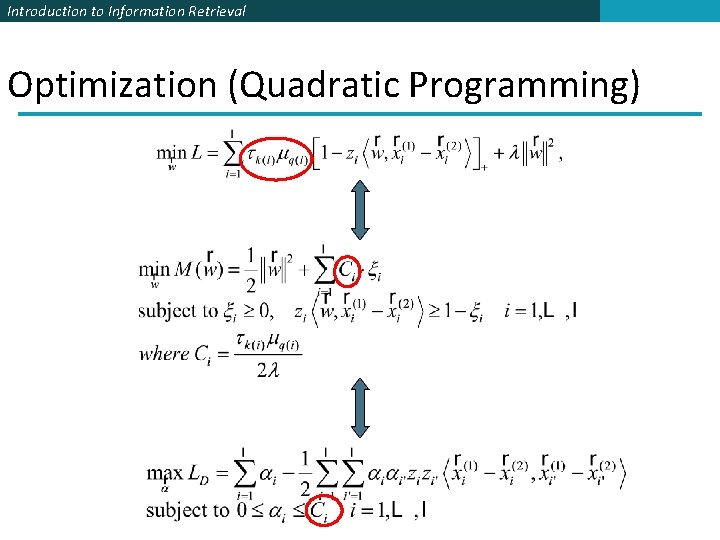Introduction to Information Retrieval Optimization (Quadratic Programming)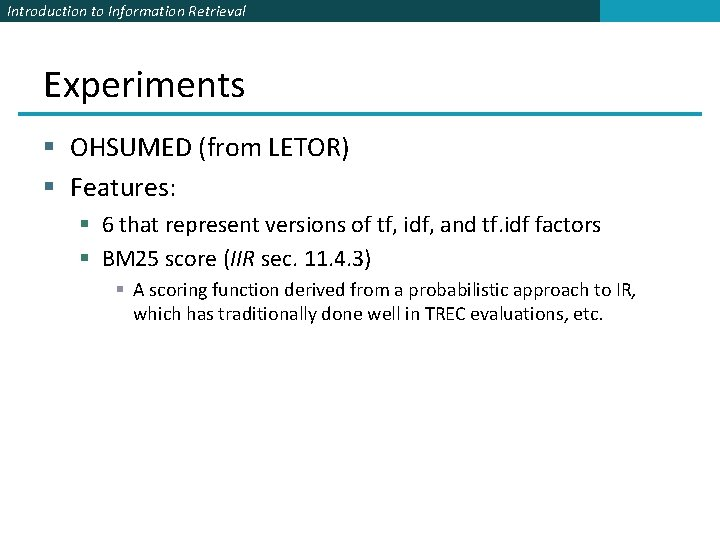Introduction to Information Retrieval Experiments § OHSUMED (from LETOR) § Features: § 6 that represent versions of tf, idf, and tf. idf factors § BM 25 score (IIR sec. 11. 4. 3) § A scoring function derived from a probabilistic approach to IR, which has traditionally done well in TREC evaluations, etc.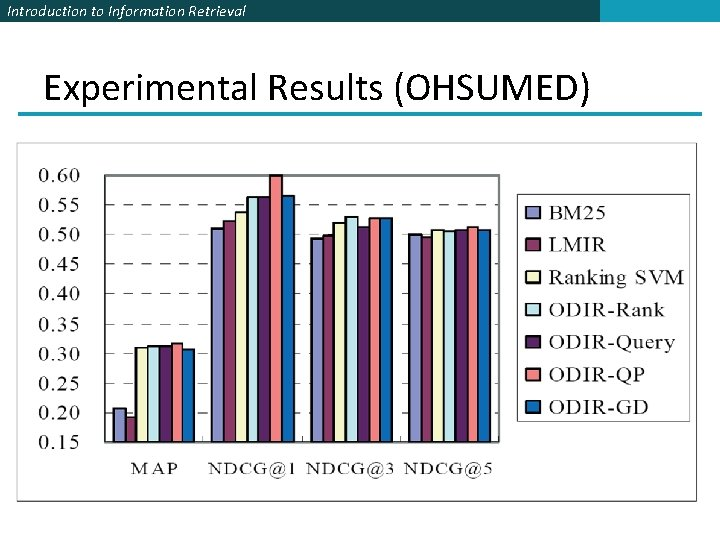Introduction to Information Retrieval Experimental Results (OHSUMED)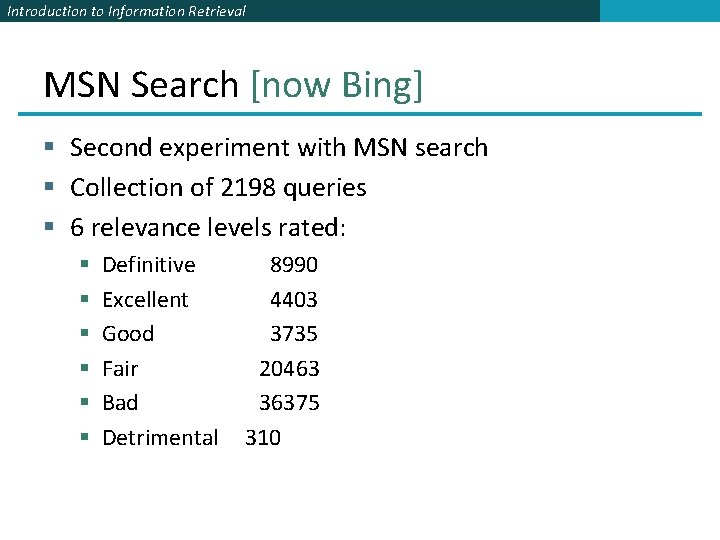Introduction to Information Retrieval MSN Search [now Bing] § Second experiment with MSN search § Collection of 2198 queries § 6 relevance levels rated: § § § Definitive Excellent Good Fair Bad Detrimental 8990 4403 3735 20463 36375 310Introduction to Information Retrieval Experimental Results (MSN search)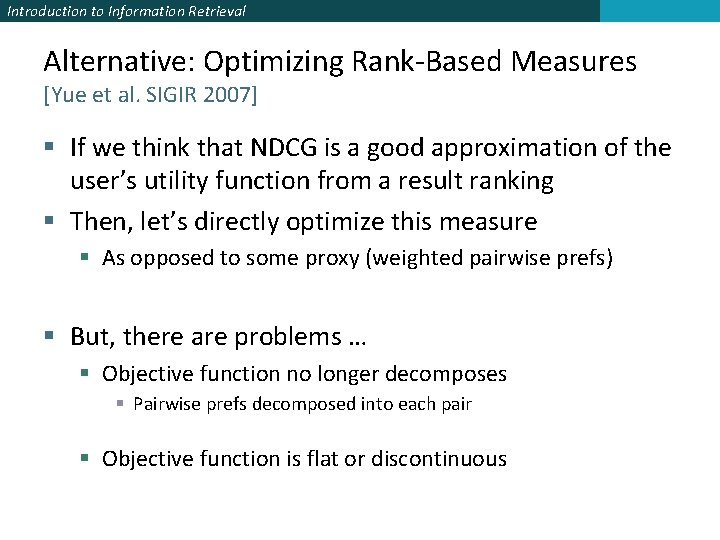Introduction to Information Retrieval Alternative: Optimizing Rank-Based Measures [Yue et al. SIGIR 2007] § If we think that NDCG is a good approximation of the user’s utility function from a result ranking § Then, let’s directly optimize this measure § As opposed to some proxy (weighted pairwise prefs) § But, there are problems … § Objective function no longer decomposes § Pairwise prefs decomposed into each pair § Objective function is flat or discontinuous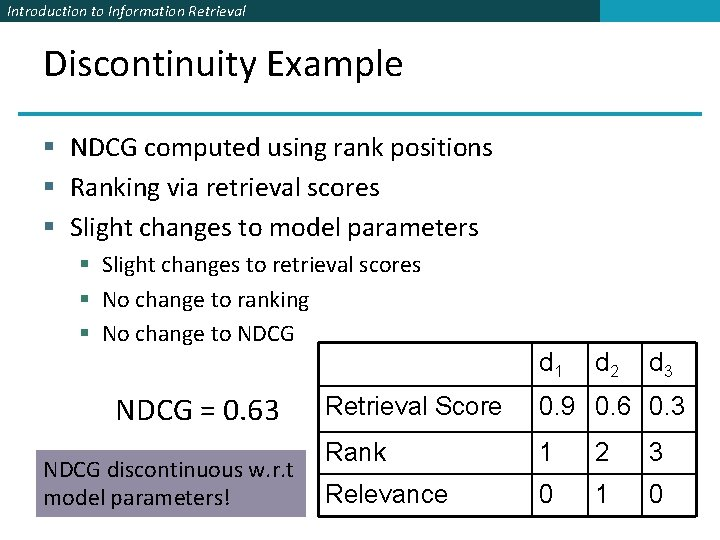Introduction to Information Retrieval Discontinuity Example § NDCG computed using rank positions § Ranking via retrieval scores § Slight changes to model parameters § Slight changes to retrieval scores § No change to ranking § No change to NDCG = 0. 63 NDCG discontinuous w. r. t model parameters! d 1 d 2 d 3 Retrieval Score 0. 9 0. 6 0. 3 Rank 1 2 3 Relevance 0 1 0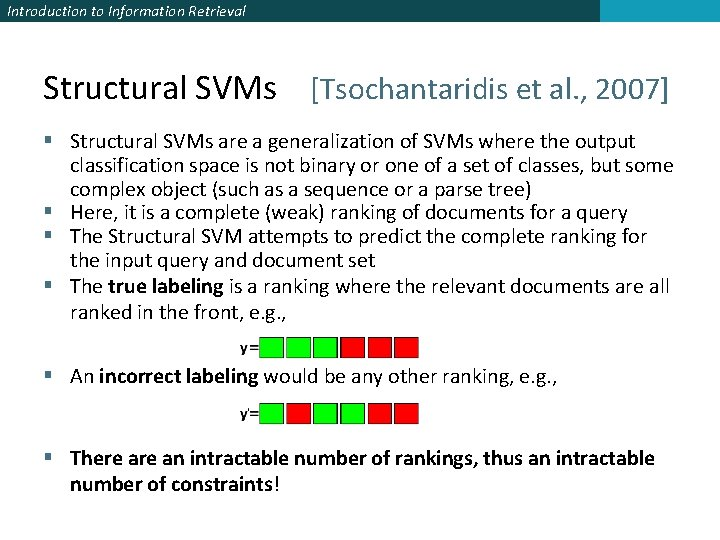Introduction to Information Retrieval Structural SVMs [Tsochantaridis et al. , 2007] § Structural SVMs are a generalization of SVMs where the output classification space is not binary or one of a set of classes, but some complex object (such as a sequence or a parse tree) § Here, it is a complete (weak) ranking of documents for a query § The Structural SVM attempts to predict the complete ranking for the input query and document set § The true labeling is a ranking where the relevant documents are all ranked in the front, e. g. , § An incorrect labeling would be any other ranking, e. g. , § There an intractable number of rankings, thus an intractable number of constraints!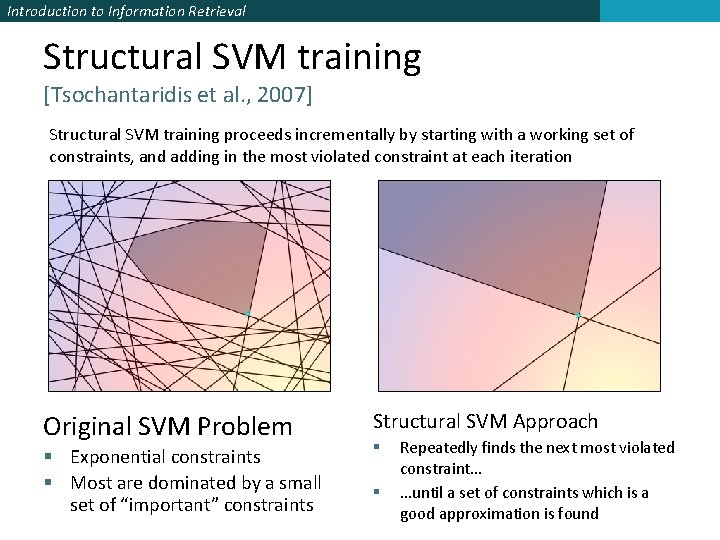Introduction to Information Retrieval Structural SVM training [Tsochantaridis et al. , 2007] Structural SVM training proceeds incrementally by starting with a working set of constraints, and adding in the most violated constraint at each iteration Original SVM Problem § Exponential constraints § Most are dominated by a small set of “important” constraints Structural SVM Approach § § Repeatedly finds the next most violated constraint… …until a set of constraints which is a good approximation is found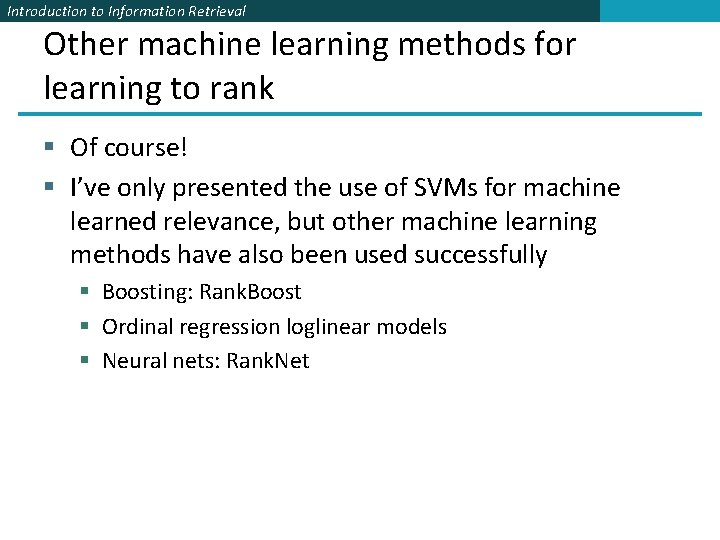Introduction to Information Retrieval Other machine learning methods for learning to rank § Of course! § I’ve only presented the use of SVMs for machine learned relevance, but other machine learning methods have also been used successfully § Boosting: Rank. Boost § Ordinal regression loglinear models § Neural nets: Rank. NetIntroduction to Information Retrieval The Limitation of Machine Learning § Everything that we have looked at (and most work in this area) produces linear models of features by weighting different base features § This contrasts with most of the clever ideas of traditional IR, which are nonlinear scalings and combinations of basic measurements § log term frequency, idf, pivoted length normalization § At present, ML is good at weighting features, but not at coming up with nonlinear scalings § Designing the basic features that give good signals for ranking remains the domain of human creativity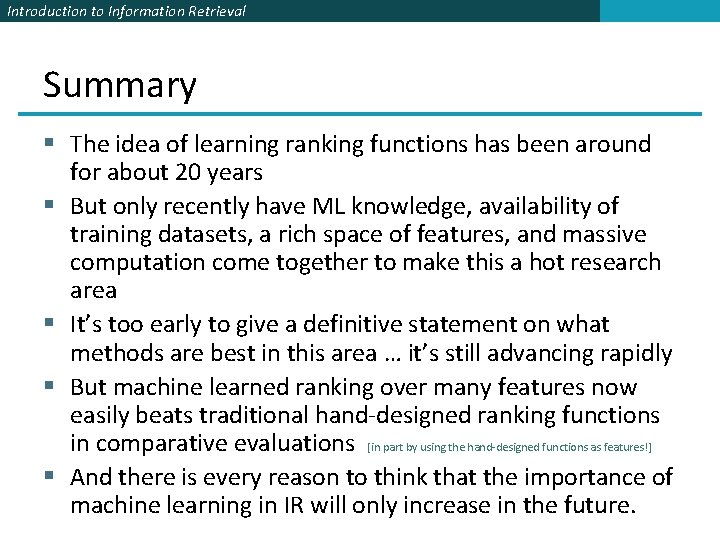Introduction to Information Retrieval Summary § The idea of learning ranking functions has been around for about 20 years § But only recently have ML knowledge, availability of training datasets, a rich space of features, and massive computation come together to make this a hot research area § It’s too early to give a definitive statement on what methods are best in this area … it’s still advancing rapidly § But machine learned ranking over many features now easily beats traditional hand-designed ranking functions in comparative evaluations [in part by using the hand-designed functions as features!] § And there is every reason to think that the importance of machine learning in IR will only increase in the future.Introduction to Information Retrieval Resources § IIR secs 6. 1. 2– 3 and 15. 4 § LETOR benchmark datasets § Website with data, links to papers, benchmarks, etc. § http: //research. microsoft. com/users/LETOR/ § Everything you need to start research in this area! § Nallapati, R. Discriminative models for information retrieval. SIGIR 2004. § Cao, Y. , Xu, J. Liu, T. -Y. , Li, H. , Huang, Y. and Hon, H. -W. Adapting Ranking SVM to Document Retrieval, SIGIR 2006. § Y. Yue, T. Finley, F. Radlinski, T. Joachims. A Support Vector Method for Optimizing Average Precision. SIGIR 2007.